# RD Sharma Solutions -Ex-19.1, Surface Area And Volume Of Right Circular Cylinder, Class 9, Maths Notes | Study RD Sharma Solutions for Class 9 Mathematics - Class 9

## Class 9: RD Sharma Solutions -Ex-19.1, Surface Area And Volume Of Right Circular Cylinder, Class 9, Maths Notes | Study RD Sharma Solutions for Class 9 Mathematics - Class 9

The document RD Sharma Solutions -Ex-19.1, Surface Area And Volume Of Right Circular Cylinder, Class 9, Maths Notes | Study RD Sharma Solutions for Class 9 Mathematics - Class 9 is a part of the Class 9 Course RD Sharma Solutions for Class 9 Mathematics.
All you need of Class 9 at this link: Class 9

Q1. Curved surface area of a right circular cylinder is 4.4m2. If the radius of the base of the cylinder is 0.7m.Find its height.(Take π=3.14)

Solution:

Given that

Radius of the base of the cylinder (r) =0.7m

Curved surface area of cylinder (C.S.A) = 4.4m2

Let the height of the cylinder be h

The curved surface area of a cylinder is given by: 2πrh

2πrh=4.4m2

2*3.14*0.7*h=4.4m2

h=4.4÷2∗3.14∗0.7

=1m

Therefore the height of the cylinder is 1m.

Q2. In a hot water heating system, there is a cylindrical pipe of length 28 m and diameter 5 cm. Find the total radiating surface in the system. (Take π=3.14)

Solution:

Given that

Height of cylinder=length of cylindrical pipe

=28m

Radius(r) of circular end of pipe=5/2=2.5cm=0.025 m

Curved surface area of cylindrical pipe=2πrh=2*3.14*0.035*30=4.4m2

Therefore the area of radiating surface of the system is 4.4mor 44000cm^{2}.

Q3. A cylindrical pillar is 50 cm in diameter and 3.5 m in height. Find the cost of painting the curved surface of the pillar at the rate of Rs 12.50 per m2.(Take   π=3.14)

Solution:

Given that

Height of cylindrical pillar=3.5m

Radius(r) of circular end of pillar=50/2=25cm=0.25m

Curved surface area of cylindrical pillar=2π=2*3.14*0.25*3.5

=5.5m2

The cost of whitewashing  1m^{2} is Rs 12.50

Cost of whitewashing 5.5m2 area=Rs(12.5*5.5) =Rs 68.75

Thus the cost of whitewashing the pillar is Rs68.75

Q4. It is required to make a closed cylindrical tank of height  1m and the base diameter of 140 cm from a metal sheet. How many square meters of the sheet are required for the same? (Take π=3.14).

Solution:

Height of the cylindrical tank(h)=1 m

Base radius of cylindricall tank(r)=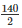=70cm=0.7m

Area of sheet required =total surface area of tank= 2πrh

=2*3.14*0.7(0.7+1)

=4.4*1.7 =7.48m2

Therefore it will require 7.48m2 of metal sheet.

Q5.A solid cylinder has a total surface area of 462cm2.Its curved surface area is one-third of its total surface area. Find the radius and height of the cylinder. (Take π=3.14).

Solution:

Given that

Curved or lateral surface area=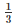* total surface area

2πrh=(2πrh+2πr2)

4πrh=2πr2

2h=r

Total surface area =462cm2

Curved surface area=13∗462

2πrh=154

2∗3.14∗2∗h2=154

h2=49/4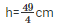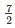cm

Now  r=2h

Therefore r=2*cm =7cm

The height and the radius of the cylinder iscm and 7 cm respectively.

Q6. The total surface area of a hollow cylinder which is open on both the sides is 4620 sq.cm and the area of the base ring is 115.5 sq.cm and height is 7cm. Find the thickness of the cylinder.

Solution:

Let the inner radius of the hollow cylinder be r1cm

The outer radius of the hollow cylinder be r2 cm

Then,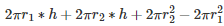=4620…………(a)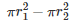=115.5……………………………………….(b)

Now solving eq(a)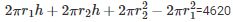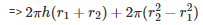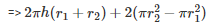Now putting the value of (b) in (a) we get

=> 2πh(r1+r2)+231=4620

=> 2π∗7(r1+r2)=4389

=> π(r1+r2)=313.5………………………………………..(c)

Now solving eq(b)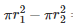=115.5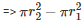=115.5

=> π(r2+r1)(r2−r1)…………………………………(d)

Dividing equation (d) by (c) we get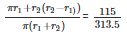=> r2−r1 =0.3684 cm

Q7. Find the ratio between the total surface area of a cylinder to its curved surface area, given that height and radius of the tank are 7.5m and 3.5 m respectively.

Solution:

Given that,

Height of the cylinder(h)=7.5m

Total Surface Area of cylinder (T.S.A)

= 2πr(r+h))

Curved surface area of a cylinder(C.S.A)

= 2πrh

Now ,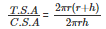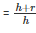……………….(1)

Putting the values in eq(1)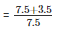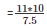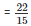= 22:15

Therefore the ratio is 22:15.

Q8.The total surface of a hollow metal cylinder, open at both ends of an external radius of 8cm and height  10cm is 338cm2.Take r to be the inner radius, obtain an equation in r and use it to obtain the thickness of the metal in the cylinder.

Solution:

Given that

The external radius of the cylinder(R)=8cm

Height of the cylinder(h)=10 cm

The total surface area of the hollow cylinder(T.S.A) = 338πcm2

As we know that,

2πr∗h+2πR∗h+2πR∗2−2πr2= 338πcm2

=> h(r+R)+(R+r)(R−r)=169

=> 10(8+r)+(8+r)(8−r)=169

=> 80+10r+64−r2=169

=> r2−10r+25=0

=> r=5

R−r=8-5cm =3cm

Q9.A cylindrical vessel, without lid, has to be tin-coated on its both sides. If the radius of the base is 70 cm and its height is 1.4m, calculate the cost of tin-coating at the rate of Rs 3.50 per 1000cm2.

Solution:

Given that

Height of  the vessel (h)=1.4m=140cm

The area to be tin coated

=2*(2πrh+πr2)

=2πr(2h+r)

= 2*3.14*70[(2*140)+70]

=154000cm2

Required cost=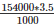=Rs 539

Q10. The inner diameter of a circular well is 3.5 m. It is 10 m deep. Find:

(a)Inner curved surface area

(b)the cost of plastering this curved surface at the rate of Rs 40 per m2.

Solution:

The inner diameter of the well=3.5m

Inner radius=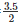=1.75m

Height of the well=10m

(a) Inner curved surface area

=2πrh

=2*3.14*1.75*10

=110m2

(b) Cost of painting 1m2 area of the well

=Rs 40

Cost of painting 110m2 area of the well

=Rs (50*110) =Rs 4400

Q11.Find the lateral surface area of a petrol storage tank is 4.2 m in diameter and 4.5 m high. How much steel was actually used, if 1/12th of the steel actually used was wasted in making the closed tank?

Solution:

I t is given that

Diameter of cylinder=4.2m

Radius of cylinder=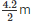=2.1m

Height of cylinder=4.5m

Therefore,

Lateral or Curved surface area= 2πrh

=2*3.14*2.1*4.5=59.4 m2

Total surface area of tank = 2*π*r(r+h)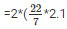(2.1+4.5) m2

= 87.12m2

Let, A m2 steel be actually used in making the tank

Area of iron present in cylinder=(y−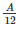m2)

=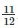Am2

Hence,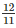A=Total surface area of cylinder

=> A=*Total surface area

=> A=(∗87.12)m2

= 95.04m2

Thus, m2 steel was actually wasted while constructing a tank.

Q12. The students of  Vidyalaya were asked to participate in a competition for making and decorating penholders in the shape of a cylinder with a base using cardboard. Each penholder was to be of radius 3 cm and height 10.5 cm. The Vidyalaya was to supply the competitors with cardboard. If there were 35 competitors, how much cardboard was required to be bought for the competition? (Take   π=3.14).

Solution:

It is given that

Radius of the circular part of the penholder (r) =3cm

The height of the penholder (h) =10.5 cm

Surface area of one penholder (S.A)

= Curved surface area of penholder +Area of the  circular base of penholder

= 2πrh+πr2

=(2*3.14*3*10.5)+3.14* 32

=198+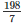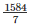cm2

The total area of cardboard sheet used by one competitor=cm2

The total area of cardboard sheet used by 30 competitors=*35 cm2

=7920cm2

Therefore, the school needs to buy 7920 cm2 of cardboard sheet for the competition.

Q13. The diameter of roller 1.5 m long is 84cm.If it takes 100 revolutions to level a playground,  find the cost of leveling this ground at the rate of 50 paise per square meter.

Solution:

Given that

Diameter of the roller (d)=85cm=0.84m

Length of the roller=1.5m

Radius of the roller(r) =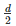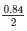=0.42m

The total area of the playground covered by the roller in one revolution=covered area of the roller

Curved surface area of the roller= 2πrh

=2*3.14*0.42*1.5

=0.12*22*1.5 m2

Area of the playground=100*Area covered by roller in one revolution

= (100*0.12*22*1.5) m2

=396 m2

Now,

Cost of leveling 1 m2=50p=Rs 0.5

Cost of leveling 396 m2 =Rs.396*0.5 =Rs.198

Hence, cost of  leveling 396m2 is Rs.198

Q14. Twenty cylindrical pillars of the Parliament House are to be cleaned. If the diameter of each pillar is 0.50m and height is 4m.What will be the cost of cleaning them at the rate of Rs 2.50 per square meter?

Solution:

Diameter of each pillar =0.5,

Radius of each pillar(r)=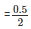=0.25m

Height of each pillar=4m

Lateral surface area of one pillar

=2πrh =2*3.14*0.25*4 =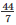m2

Lateral surface area of 20 pillars=20*m2

Cost of cleaning one pillar =Rs2.50 per square meter

Cost of cleaning 20 pillars =Rs 2.50 *20*m2 =Rs. 314.28

The document RD Sharma Solutions -Ex-19.1, Surface Area And Volume Of Right Circular Cylinder, Class 9, Maths Notes | Study RD Sharma Solutions for Class 9 Mathematics - Class 9 is a part of the Class 9 Course RD Sharma Solutions for Class 9 Mathematics.
All you need of Class 9 at this link: Class 9Use Code STAYHOME200 and get INR 200 additional OFF

## RD Sharma Solutions for Class 9 Mathematics

91 docs

Track your progress, build streaks, highlight & save important lessons and more!

,

,

,

,

,

,

,

,

,

,

,

,

,

,

,

,

,

,

,

,

,

,

,

,

,

,

,

,

,

,

;